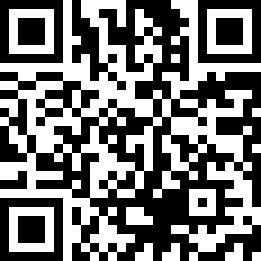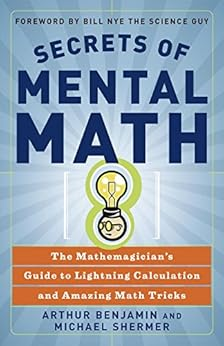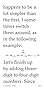## 购买选项

 Kindle电子书价格: ￥87.55# Secrets of Mental Math: The Mathemagician's Guide to Lightning Calculation and Amazing Math Tricks (English Edition) Kindle电子书

 /* * Fix for UDP-1061. Average customer reviews has a small extra line on hover * https://omni-grok.amazon.com/xref/src/appgroup/websiteTemplates/retail/SoftlinesDetailPageAssets/udp-intl-lock/src/legacy.css?indexName=WebsiteTemplates#40 */ .noUnderline a:hover { text-decoration: none; }

|

 亚马逊价格 全新品最低价 非全新品最低价
 Kindle电子书 "请重试" ￥87.55 — —
 页数 : 生词提示功能: 更先进的排版模式: 快速翻书: 语种： 英语
• 由于文件较大，下载时间可能较长。

## 商品描述

### 媒体推荐

“A great introduction to the wonder of numbers, from two superb teachers.”
—Brian Greene, author of The Elegant Universe

“A magical mystery tour of mental mathematics! Fascinating and fun.”
—Joseph Gallian, president of the Mathematical Association of America

“The clearest, simplest, most entertaining, and best book yet on the art of calculating in your head.”
—Martin Gardner, author of Mathematical Magic Show and Mathematical Carnival

“This book can teach you mental math skills that will surprise you and your friends. Better, you will have fun and have valuable practical tools inside your head.”
—Dr. Edward O. Thorp, mathematician and author of Beat the Dealer and Beat the Market --此文字指其他 kindle_edition 版本。

### 文摘

Chapter 0

Quick Tricks: Easy (and Impressive) Calculations

In the pages that follow, you will learn to do math in your head faster than you ever thought possible. After practicing the methods in this book for just a little while, your ability to work with numbers will increase dramatically. With even more practice, you will be able to perform many calculations faster than someone using a calculator. But in this chapter, my goal is to teach you some easy yet impressive calculations you can learn to do immediately. We’ll save some of the more serious stuff for later.

Instant Multiplication

Let’s begin with one of my favorite feats of mental math—how to multiply, in your head, any two-digit number by eleven. It’s very easy once you know the secret. Consider the problem:

32 3 11

To solve this problem, simply add the digits, 3 1 2 5 5}, put the 5 between the 3 and the 2, and there is your answer:

35}2

What could be easier? Now you try:

53 3 11

583

One more. Without looking at the answer or writing anything down, what is

81 3 11?

Did you get 891? Congratulations!

Now before you get too excited, I have shown you only half of what you need to know. Suppose the problem is

85 3 11

Although 8 1 5 5 1}3}, the answer is NOT 81}3}5!

As before, the 3} goes in between the numbers, but the 1} needs to be added to the 8 to get the correct answer:

93}5

Think of the problem this way:

Here is another example. Try 57 3 11.

Since 5 1 7 5 12, the answer is

Okay, now it’s your turn. As fast as you can, what is

77 3 11?

If you got the answer 847, then give yourself a pat on the back. You are on your way to becoming a mathemagician.

Now, I know from experience that if you tell a friend or teacher that you can multiply, in your head, any two-digit number by eleven, it won’t be long before they ask you to do 99 3 11. Let’s do that one now, so we are ready for it.

Since 9 1 9 5 18, the answer is:

Okay, take a moment to practice your new skill a few times, then start showing off. You will be amazed at the reaction you get. (Whether or not you decide to reveal the secret is up to you!)

Welcome back. At this point, you probably have a few questions, such as:

“Can we use this method for multiplying three-digit numbers (or larger) by eleven?”

Absolutely. For instance, for the problem 314 3 11, the answer still begins with 3 and ends with 4. Since 3 1 1 5 4}, and 1 1 4 5 5}, the answer is 34}5}4. But we’ll save larger problems like this for later.

More practically, you are probably saying to yourself,

“Well, this is fine for multiplying by elevens, but what about larger numbers? How do I multiply numbers by twelve, or thirteen, or thirty-six?”

My answer to that is, Patience! That’s what the rest of the book is all about. In Chapters 2, 3, 6, and 8, you will learn methods for multiplying together just about any two numbers. Better still, you don’t have to memorize special rules for every number. Just a handful of techniques is all that it takes to multiply numbers in your head, quickly and easily.

Squaring and More

Here is another quick trick.

As you probably know, the square of a number is a number multiplied by itself. For example, the square of 7 is 7 3 7 5 49. Later, I will teach you a simple method that will enable you to easily calculate the square of any two-digit or three-digit (or higher) number. That method is especially simple when the number ends in 5, so let’s do that trick now.

To square a two-digit number that ends in 5, you need to remember only two things.

1.The answer begins by multiplying the first digit by the next higher digit.

For example, to square the number 35, we simply multiply the first digit (3) by the next higher digit (4), then attach 25. Since 3 3 4 5 12, the answer is 1225. Therefore, 35 3 35 5 1225. Our steps can be illustrated this way:

How about the square of 85? Since 8 3 9 5 72, we immediately get 85 3 85 5 7225.

We can use a similar trick when multiplying two-digit numbers with the same first digit, and second digits that sum to 10. The answer begins the same way that it did before (the first digit multiplied by the next higher digit), followed by the product of the second digits. For example, let’s try 83 3 87. (Both numbers begin with 8, and the last digits sum to 3 1 7 5 10.) Since 8 3 9 5 72, and 3 3 7 5 21, the answer is 7221.

Similarly, 84 3 86 5 7224.

26 3 24

How does the answer begin? With 2 3 3 5 6. How does it end? With 6 3 4 5 24. Thus 26 3 24 5 624.

Remember that to use this method, the first digits have to be the same, and the last digits must sum to 10. Thus, we can use this method to instantly determine that

31 3 39 5 1209

32 3 38 5 1216

33 3 37 5 1221

34 3 36 5 1224

35 3 35 5 1225

“What if the last digits do not sum to ten? Can we use this method to multiply twenty-two and twenty-three?”

Well, not yet. But in Chapter 8, I will show you an easy way to do problems like this using the close-together method. (For 22 3 23, you would do 20 3 25 plus 2 3 3, to get 500 1 6 5 506, but I’m getting ahead of myself!) Not only will you learn how to use these methods, but you will understand why these methods work, too.

“Are there any tricks for doing mental addition and subtraction?”

Definitely, and that is what the next chapter is all about. If I were forced to summarize my method in three words, I would say, “Left to right.” Here is a sneak preview.

Consider the subtraction problem

Most people would not like to do this problem in their head (or even on paper!), but let’s simplify it. Instead of subtracting 587, subtract 600. Since 1200 2 600 5 600, we have that

But we have subtracted 13 too much. (We will explain how to quickly determine the 13 in Chapter 1.) Thus, our painful-looking subtraction problem becomes the easy addition problem

which is not too hard to calculate in your head (especially from left to right). Thus, 1241 2 587 5 654.

Using a little bit of mathematical magic, described in Chapter 9, you will be able to instantly compute the sum of the ten numbers below.

Although I won’t reveal the magical secret right now, here is a hint. The answer, 935, has appeared elsewhere in this chapter. More tricks for doing math on paper will be found in Chapter 6. Furthermore, you will be able to quickly give the quotient of the last two numbers:

359 4 222 5 1.61 (first three digits)

We will have much more to say about division (including decimals and fractions) in Chapter 4.

More Practical Tips

Here’s a quick tip for calculating tips. Suppose your bill at a restaurant came to \$42, and you wanted to leave a 15% tip. First we calculate 10% of \$42, which is \$4.20. If we cut that number in half, we get \$2.10, which is 5% of the bill. Adding these numbers together gives us \$6.30, which is exactly 15% of the bill. We will discuss strategies for calculating sales tax, discounts, compound interest, and other practical items in Chapter 5, along with strategies that you can use for quick mental estimation when an exact answer is not required.

In Chapter 7, you will learn a useful technique for memorizing numbers. This will be handy in and out of the classroom. Using an easy-to-learn system for turning numbers into words, you will be able to quickly and easily memorize any numbers: dates, phone numbers, whatever you want.

Speaking of dates, how would you like to be able to figure out the day of the week of any date? You can use this to figure out birth dates, historical dates, future appointments, and so on. I will show you this in more detail later, but here is a simple way to figure out the day of January 1 for any year in the twenty-first century. First familiarize yourself with the following table.

MondayTuesdayWednesdayThursdayFridaySaturdaySunday

1234567 or 0

For instance, let’s determine the day of the week of January 1, 2030. Take the last two digits of the year, and consider it to be your bill at a restaurant. (In this case, your bill would be \$30.) Now add a 25% tip, but keep the change. (You can compute this by cutting the bill in half twice, and ignoring any change. Half of \$30 is \$15. Then half of \$15 is \$7.50. Keeping the change results in a \$7 tip.) Hence your bill plus tip amounts to \$37. To figure out the day of the week, subtract the biggest multiple of 7 (0, 7, 14, 21, 28, 35, 42, 49, . . .) from your total, and that will tell you the day of the week. In this case, 37 2 35 5 2, and so January 1, 2030, will occur on 2’s day, namely Tuesday:

Bill:30

Tip: 1} } }7}

37

subtract 7s: 2} }3}5}

2 5 Tuesday

Bill:43

Tip: 1} }1}0}

53

subtract 7s: 2} }4}9}

4 5 Thursday

Exception: If the year is a leap year, remove \$1 from your tip, then proceed as before. For example, for January 1, 2032, a 25% tip of \$32 would be \$8. Removing one dollar gives a total of

32 1 7 5 39. Subtracting the largest multiple of 7 gives us 39 2 35 5 4. So January 1, 2032, will be on 4’s day, namely Thursday. For more details that will allow you to compute the day of the week of any date in history, see Chapter 9. (In fact, it’s perfectly okay to read that chapter first!)

I know what you are wondering now:

“Why didn’t they teach this to us in school?”

I’m afraid that there are some questions that even I cannot answer. Are you ready to learn more magical math? Well, what are we waiting for? Let’s go!

Chapter 1

A Little Give and Take:

For as long as I can remember, I have always found it easier to add and subtract numbers from left to right instead of from right to left. By adding and subtracting numbers this way, I found that I could call out the answers to math problems in class well before my classmates put down their pencils. And I didn’t even need a pencil!

In this chapter you will learn the left-to-right method of doing mental addition and subtraction for most numbers that you encounter on a daily basis. These mental skills are not only important for doing the tricks in this book but are also indispensable in school, at work, or any time you use numbers. Soon you will be able to retire your calculator and use the full capacity of your mind as you add and subtract two-digit, three-digit, and even four-digit numbers with lightning speed.

With the first set of problems—two-digit addition—the left-to-right method may not seem so advantageous. But be patient. If you stick with me, you will see that the only easy way to solve three-digit and larger addition problems, all subtraction problems, and most definitely all multiplication and division problems is from left to right. The sooner you get accustomed to computing this way, the better.

Our assumption in this chapter is that you know how to add and subtract one-digit numbers. We will begin with two-digit addition, something I suspect you can already do fairly well in your head. The following exercises are good practice, however, because the two-digit addition skills that you acquire here will be needed for larger addition problems, as well as virtually all multiplication problems in later chapters. It also illustrates a fundamental principle of mental arithmetic—namely, to simplify your problem by breaking it into smaller, more manageable parts. This is the key to virtually every method you will learn in this book. To paraphrase an old saying, there are three components to success—simplify, simplify, simplify.

The easiest two-digit addition problems are those that do not require you to carry any numbers, when the first digits sum to 9 or below and the last digits sum to 9 or below. For example:

(30 1 2)

To solve 47 1 32, first add 30, then add 2. After adding 30, you have the simpler problem 77 1 2, which equals 79. We illustrate this as follows:

47 1 32   5   77 1 2   5   79

The above diagram is simply a way of representing the mental processes involved in arriving at an answer using our method. While you need to be able to read and understand such diagrams as you work your way through this book, our method does not require you to write down anything yourself.
--此文字指其他 kindle_edition 版本。

## 基本信息

• ASIN ‏ : ‎ B000Q80SM6
• 出版社 ‏ : ‎ Crown (2008年6月3日)
• 出版日期 ‏ : ‎ 2008年6月3日
• 语言 ‏ : ‎ 英语
• 文件大小 ‏ : ‎ 126730 KB
• 标准语音朗读 ‏ : ‎ 已启用
• X-Ray ‏ : ‎ 已启用
• 生词提示功能 ‏ : ‎ 已启用
• 纸书页数 ‏ : ‎ 296页
• 用户评分:

## 买家评论

5.0 颗星，最多 5 颗星
5星，共 5 星
1 买家评价
 4 星 0% (0%) 0% 3 星 0% (0%) 0% 2 星 0% (0%) 0% 1 星 0% (0%) 0%

### 此商品在美国亚马逊上最有用的商品评论

S.D.
2.0 颗星，最多 5 颗星 Mixed Bag
2017年3月25日 - 已在美国亚马逊上发表

169 个人发现此评论有用
Jim Romo
5.0 颗星，最多 5 颗星 Who Knew Math Could Be FUN?
2018年4月3日 - 已在美国亚马逊上发表

32 个人发现此评论有用
Aaron Ziegler
4.0 颗星，最多 5 颗星 Should be given to all elementary school students.
2016年6月1日 - 已在美国亚马逊上发表

42 个人发现此评论有用
darkguardian2
5.0 颗星，最多 5 颗星 Good Book...Bad Kindle Format
2019年6月5日 - 已在美国亚马逊上发表5.0 颗星，最多 5 颗星 Good Book...Bad Kindle Format
2019年6月4日 在美国审核
This is a good math book that easy to read and very motivating.
It's similar to other books about math like "Math Magic" by Scott Flansburg (The Human Calculator).
I issue is the Kindle format having the examples set to small print.
Enlarging the letters doesn't help and disrupts the flow of reading the material. (Pictured)
Since, it's a math book the examples are critical to understanding the concepts.
I highly recommend it and no in-place advertisements in the texts hawking up training packages.7 个人发现此评论有用
Tom Steele
5.0 颗星，最多 5 颗星 Awesome book that shows the beauty of math...
2016年10月31日 - 已在美国亚马逊上发表

77 个人发现此评论有用